# Basic Set Notation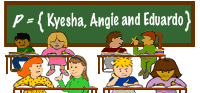Problem 1: Mrs. Glosser asked Kyesha, Angie and Eduardo to join the new math club. After school they signed up and became members. They wrote about it on the chalkboard using set notation:

P = {Kyesha, Angie and Eduardo}

When Angie's mother came to pick her up, she looked at the chalkboard and asked: What does that mean?

Solution: Let P be the set of all members in the math club.

An object that belongs to a set is called an element (or a member) of that set. We use special notation to indicate whether or not an element belongs to a set, as shown below.

 Symbol Meaningis an element of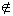is not an element of

For example, given the set A = {1, 2, 3, 4, 5}, we can write 1A, which stands for 1 is an element of set A. We can also write 7A, which stands for 7 is not an element of set A. Let's look at some more examples of this.

 Set Notation Meaning A = {2, 4, 6, 8} 2A 2 is an element of A 5A 5 is not an element of A B = {a, e, i, o, u} eB e is an element of B wB w is not an element of B C = {1, 3, 5, 7, 9} 7C 7 is an element of C 2C 2 is not an element of C D = {-3, -2, -1, 0, 1, 2, 3}-2 is an element of D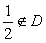One-half is not an element of D
 Determine if the given item is an element of the set. Set Item Is an element? R = {2, 4, 6, 8} 10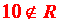S = {2, 4, 6, 8, 10} 10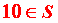D = {English alphabet} mD = {English alphabet}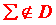X = {prime numbers less than 10} 9A = {even numbers} 8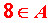Summary: An object that belongs to a set is called an element (or a member) of that set. We use special notation to indicate whether or not an element belongs to a set: (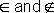),

### Exercises

 1. Which of the following is a true statement for set R?  R = {liquid, gas, solid, plasma} gas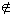R solidR liquid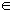R None of the above. RESULTS BOX:
 2. Which of the following is true for set G? G = {1, 3, 5, 7, 9} 5G 7G 3G All of the above. RESULTS BOX:
 3. Which of the following statements is true about set B? B = {US flag colors} redB blueB whiteB All of the above. RESULTS BOX: